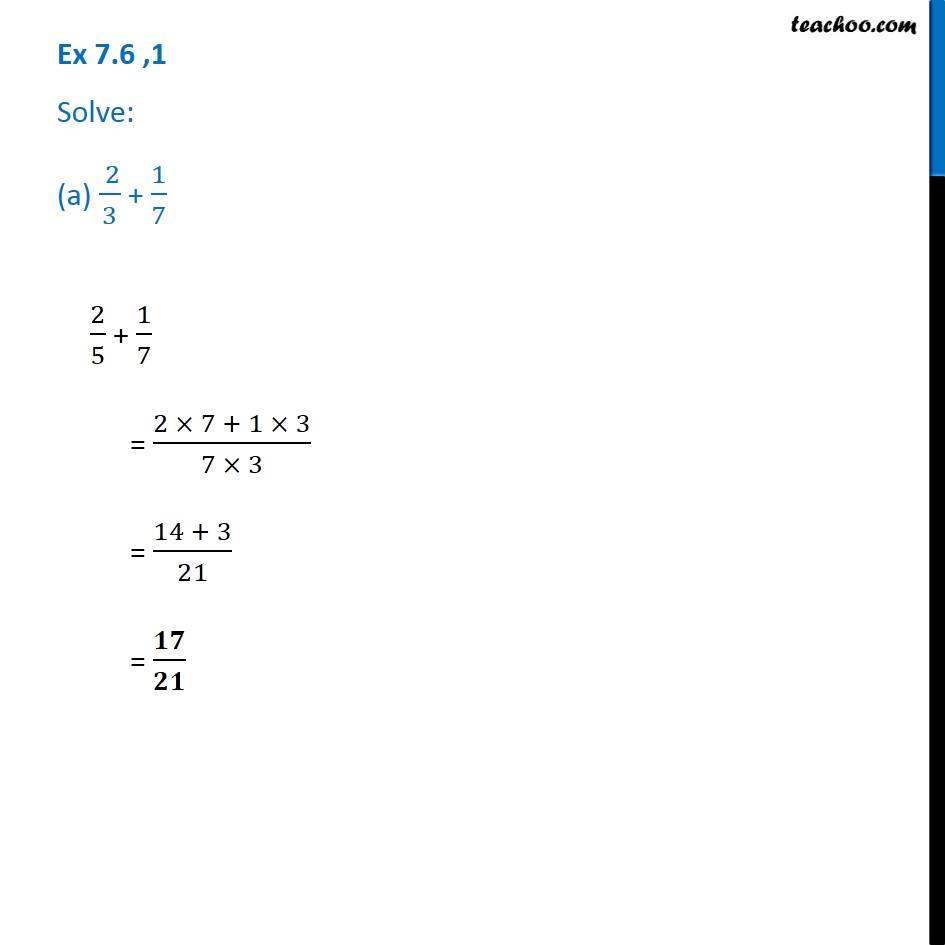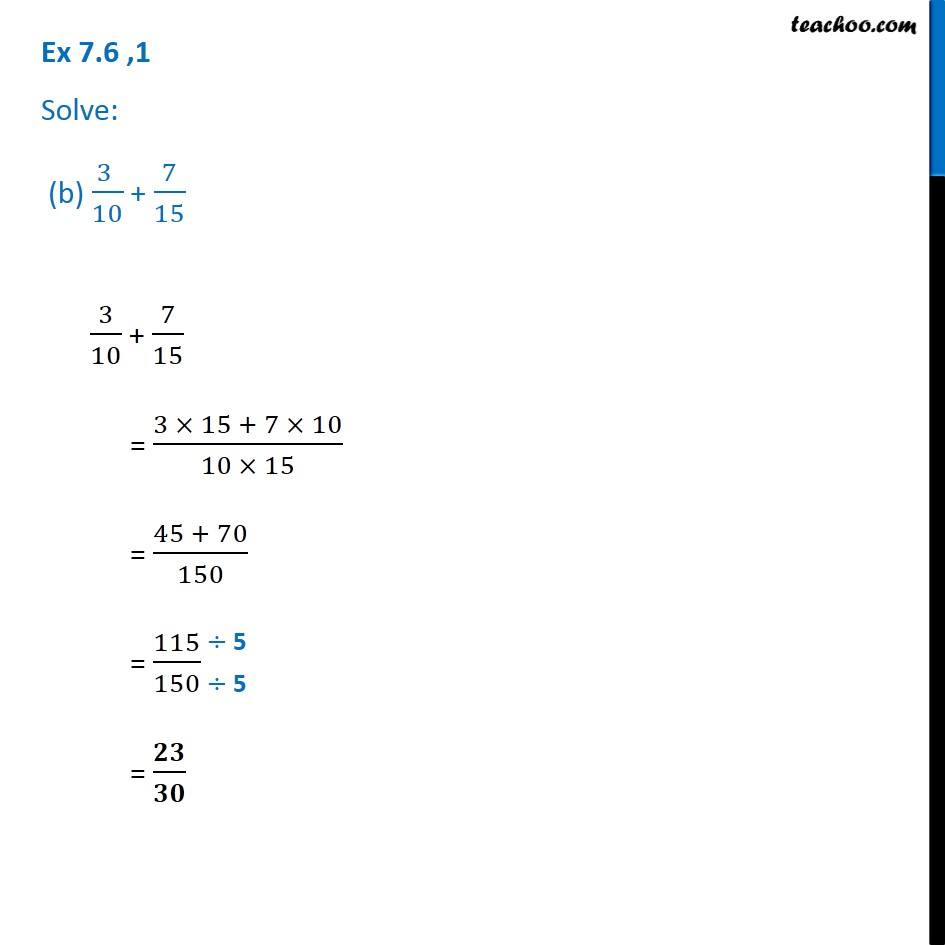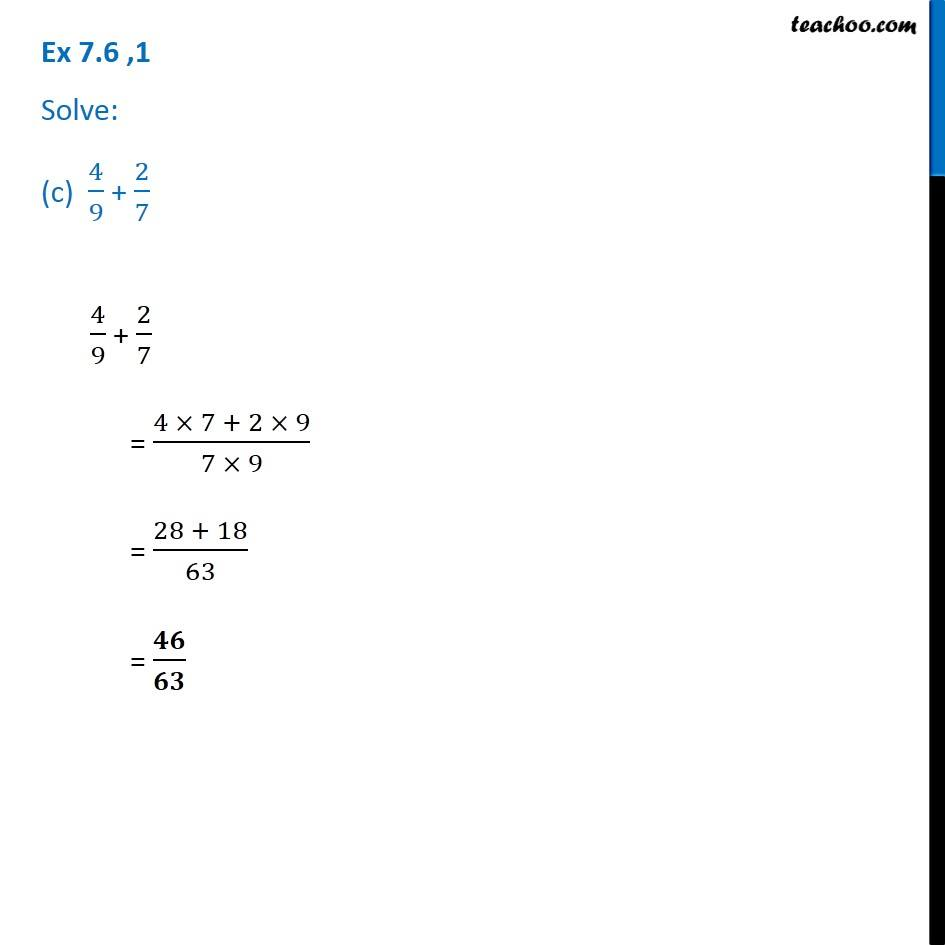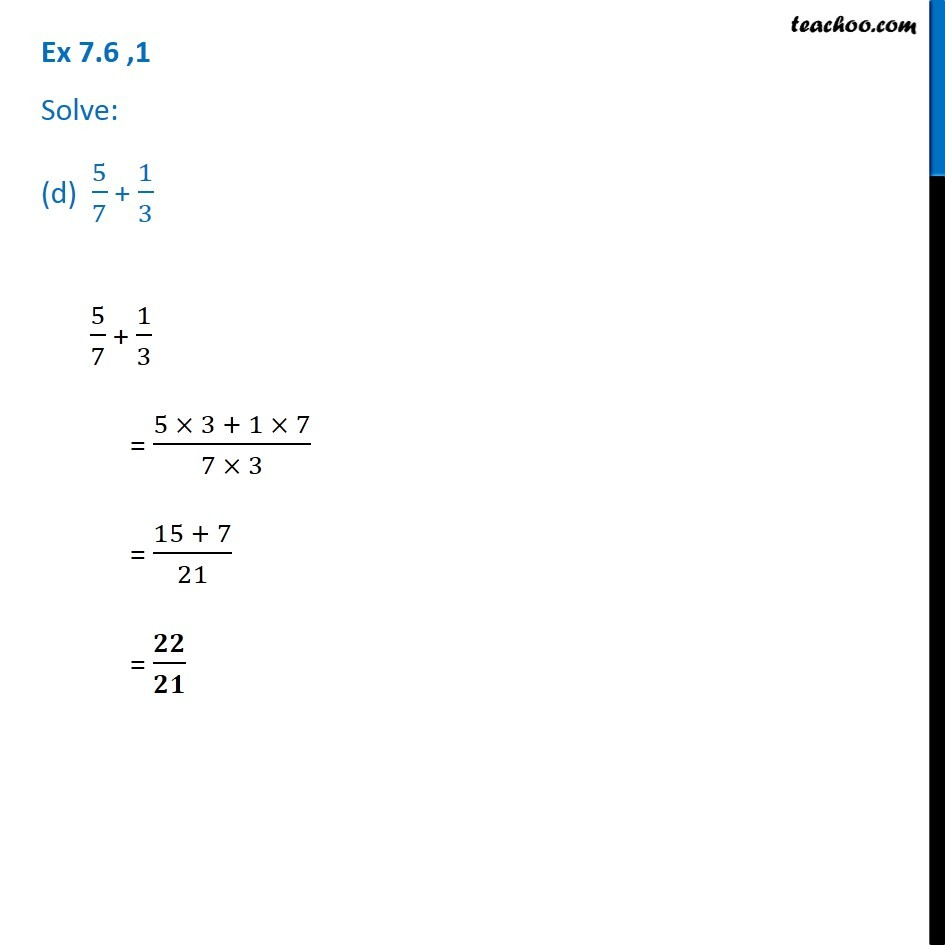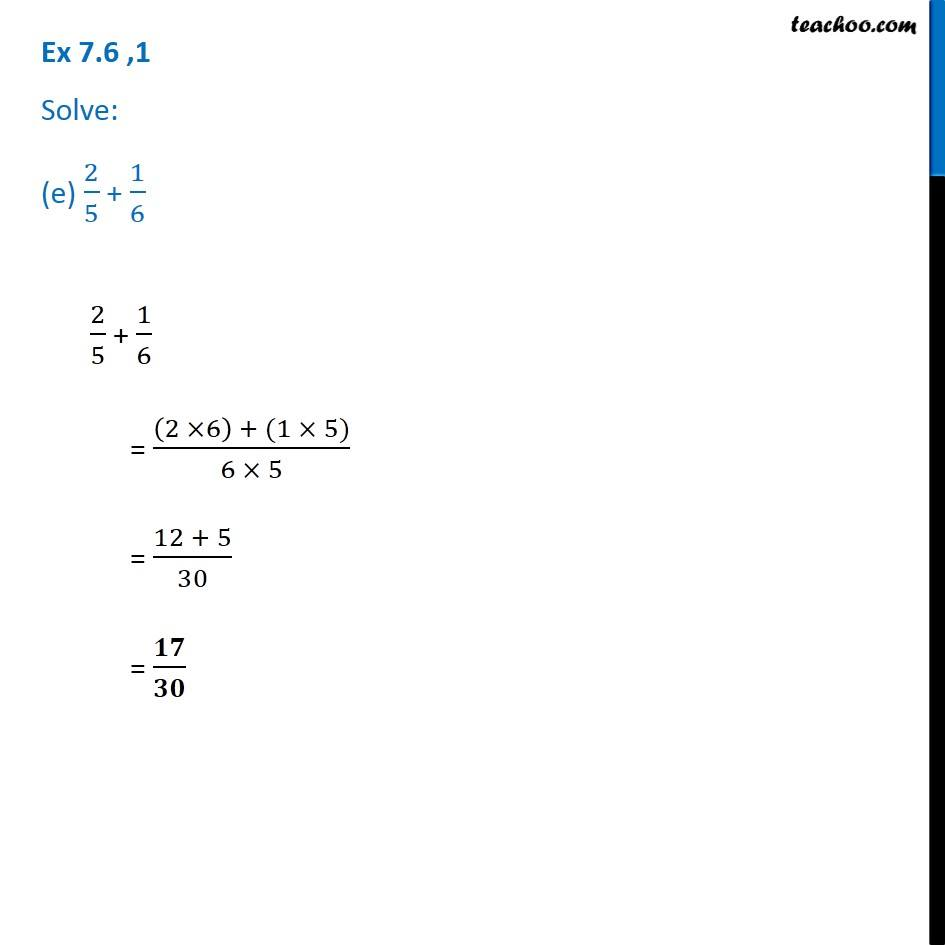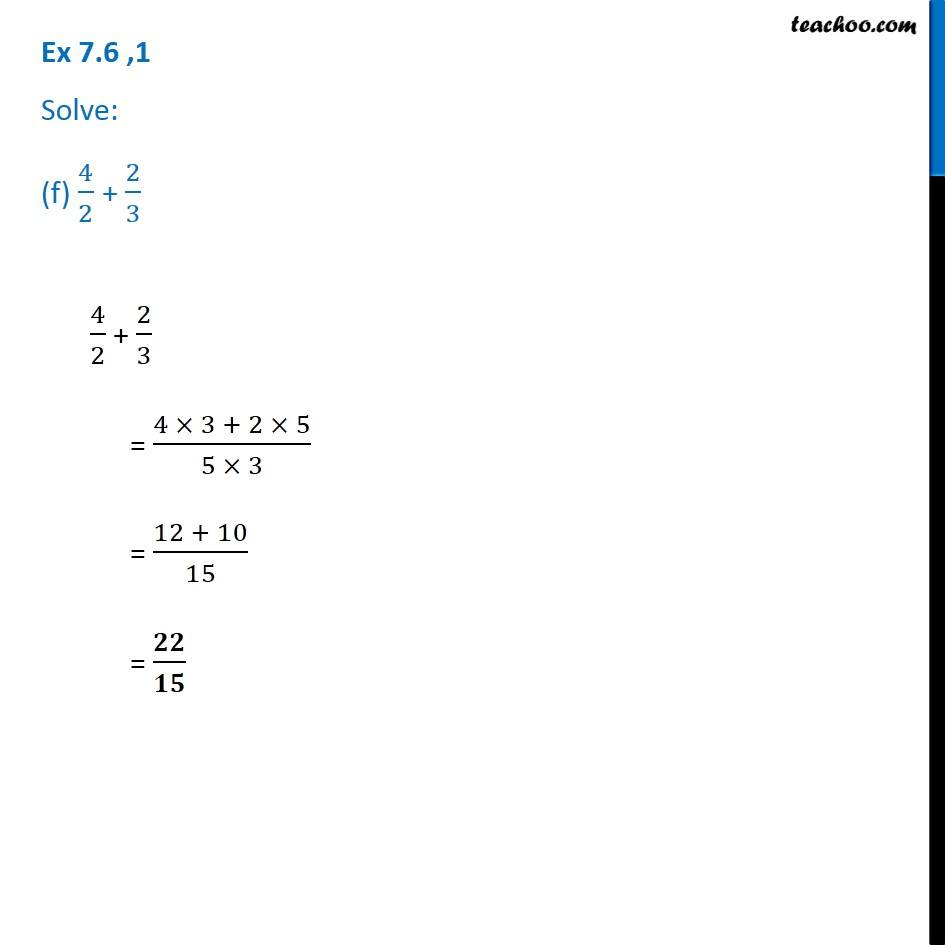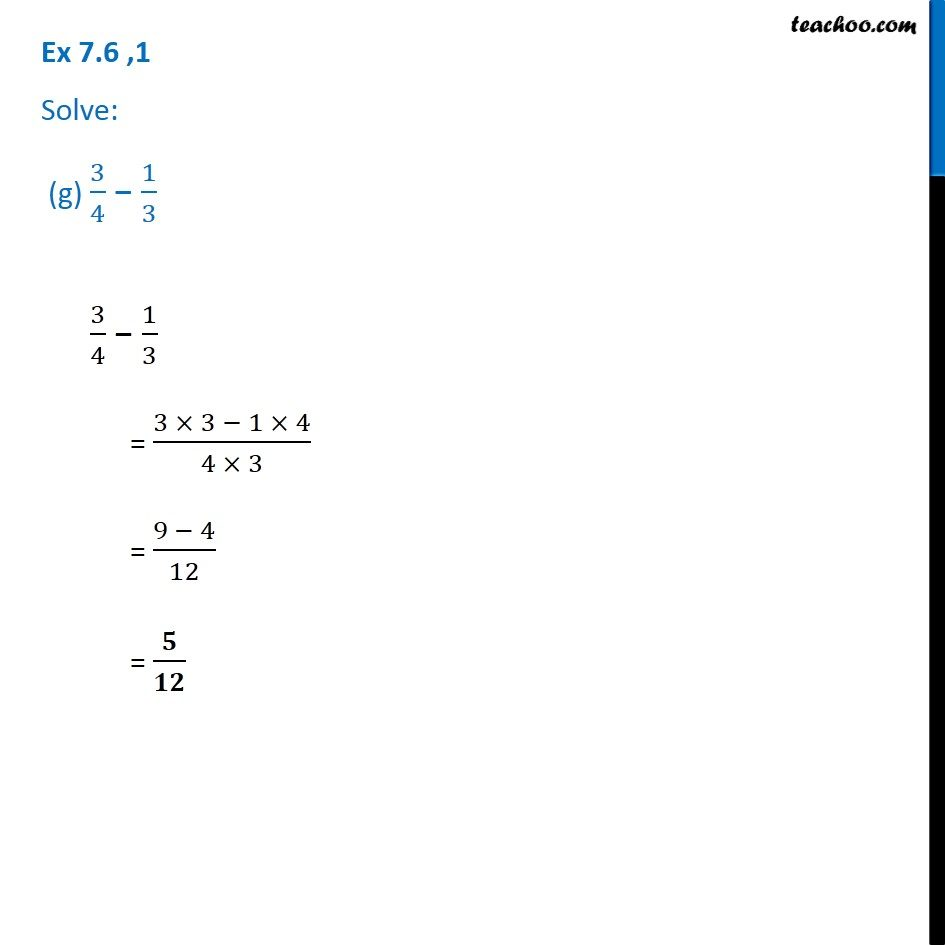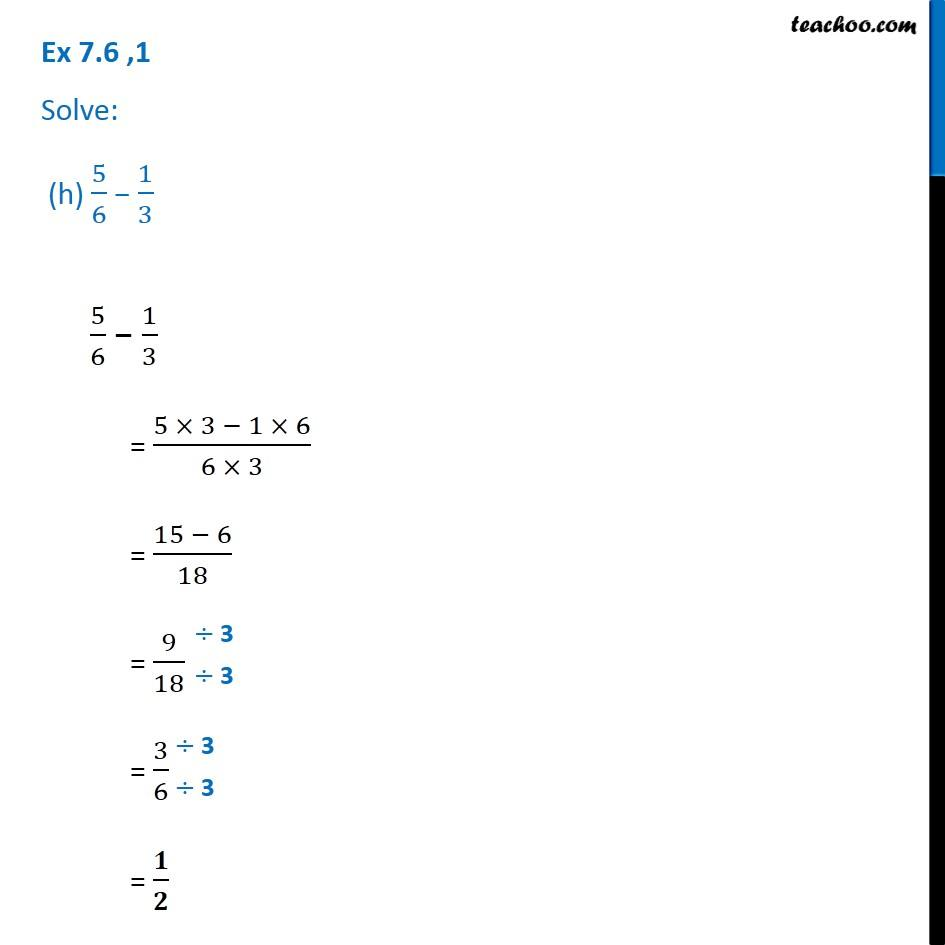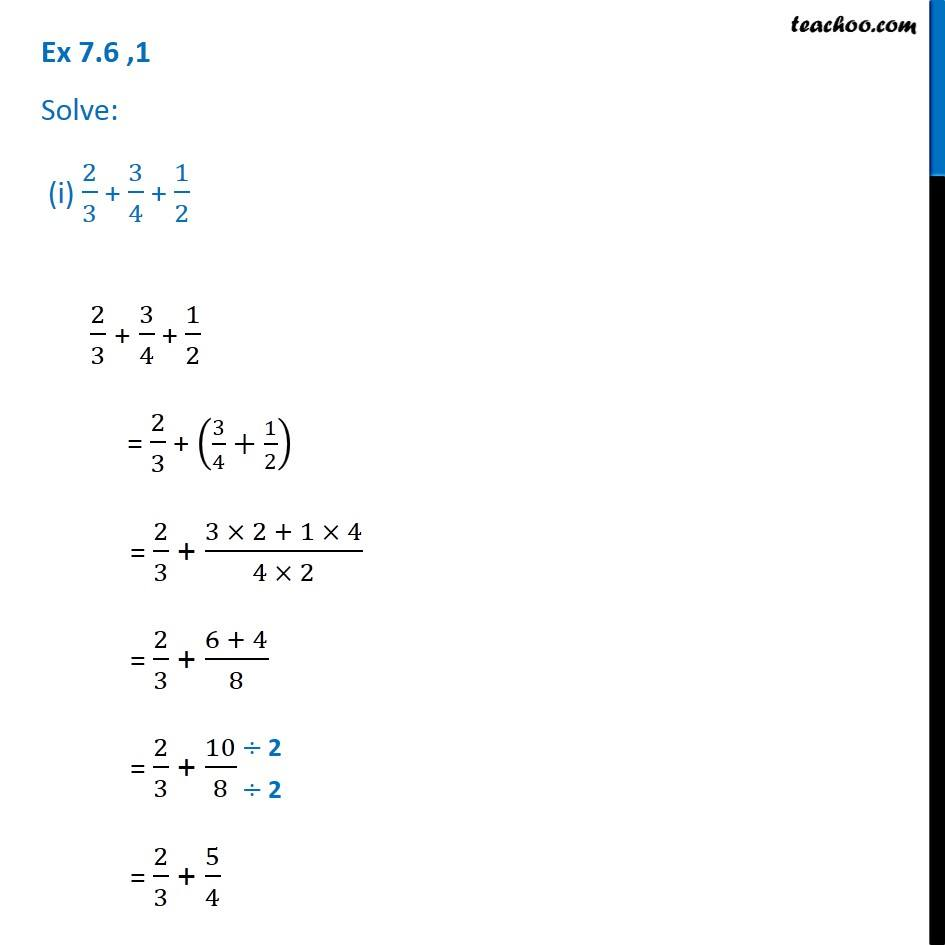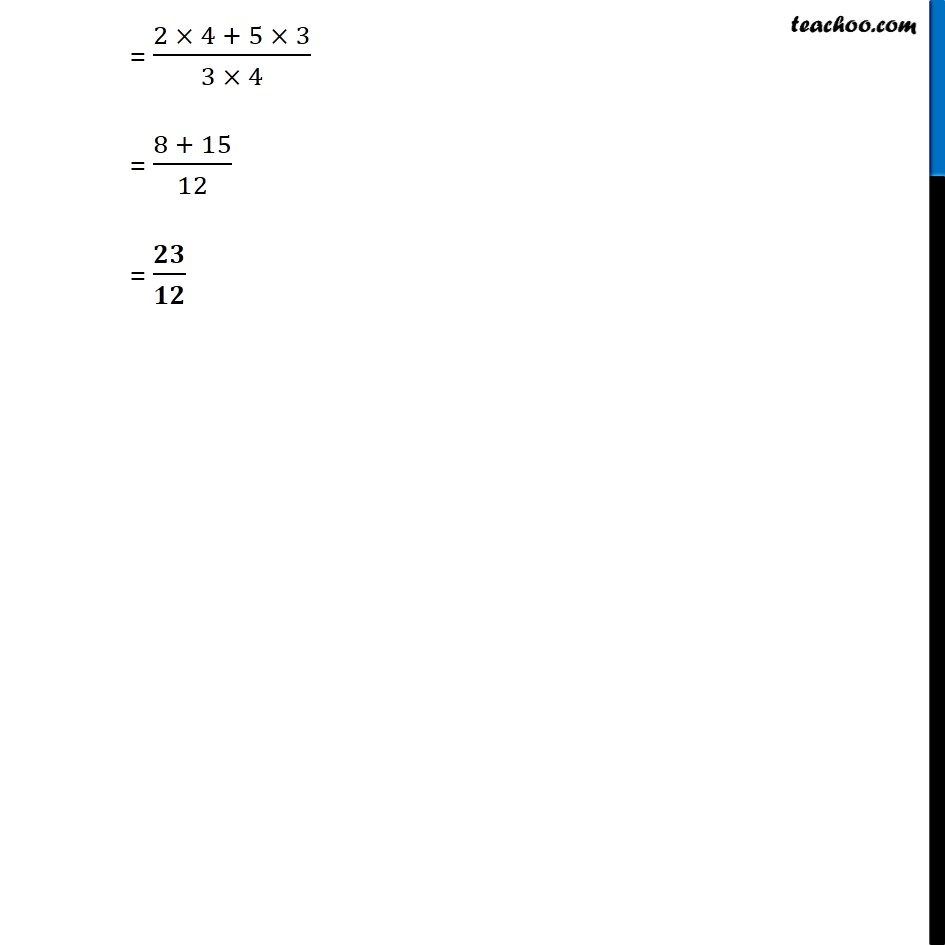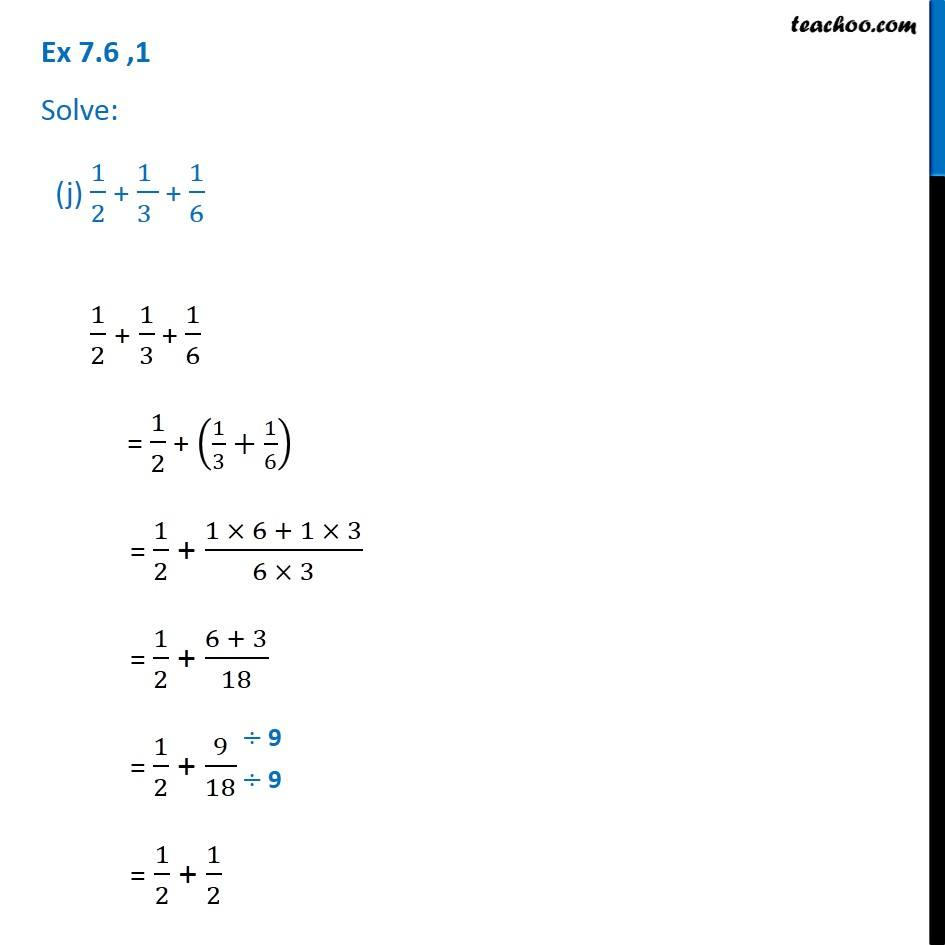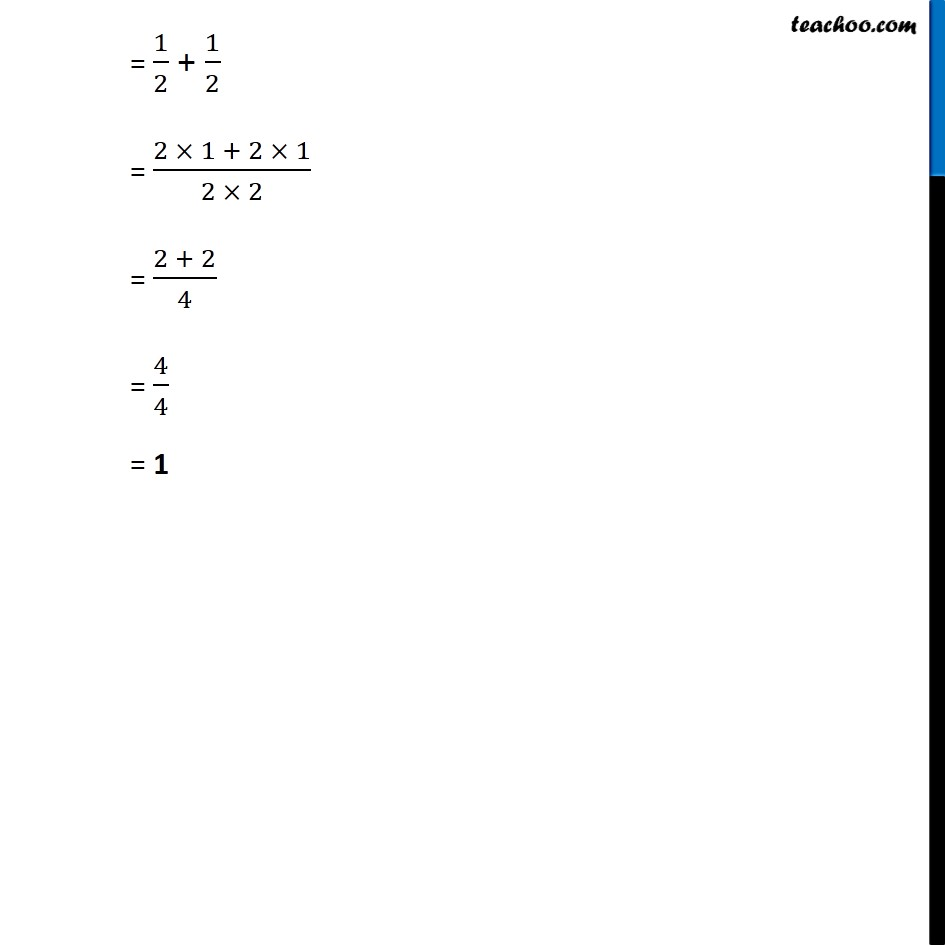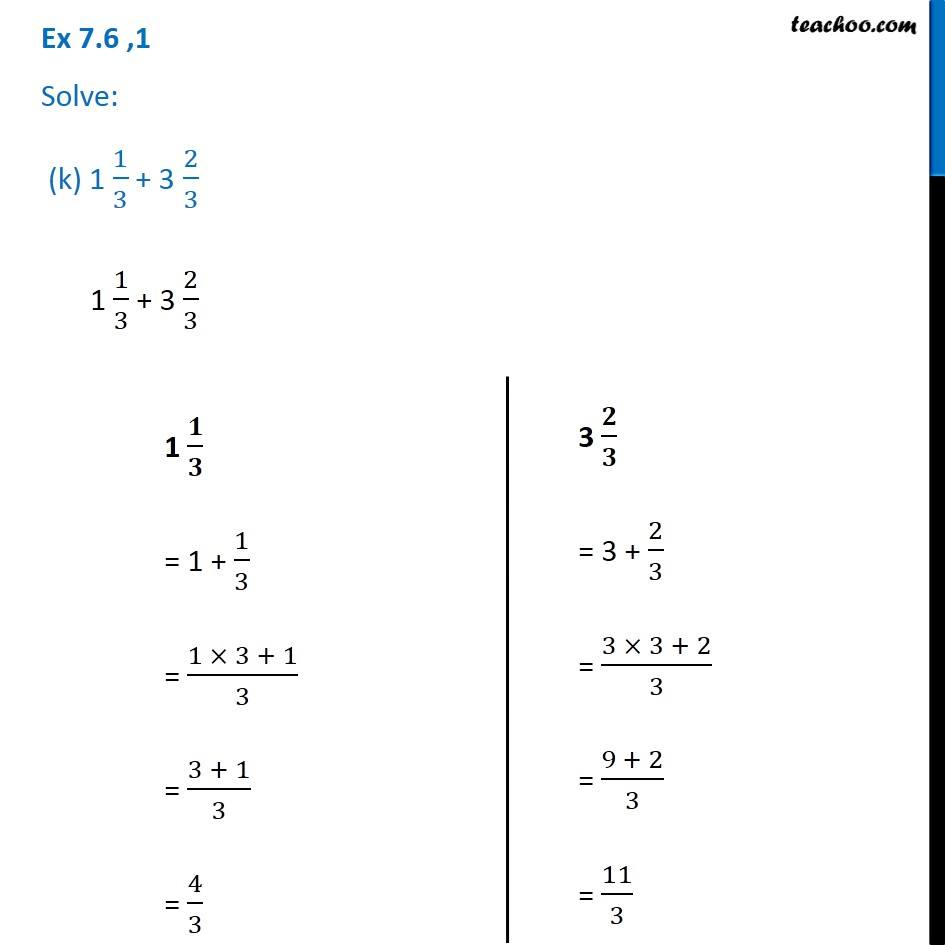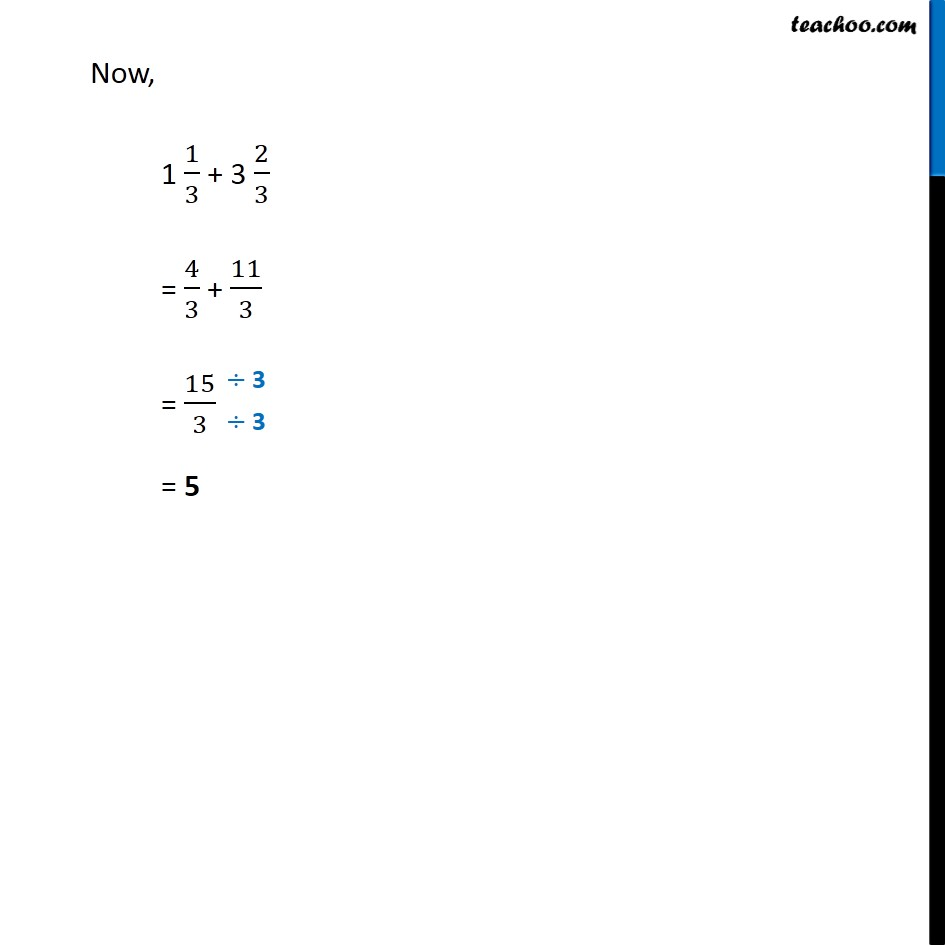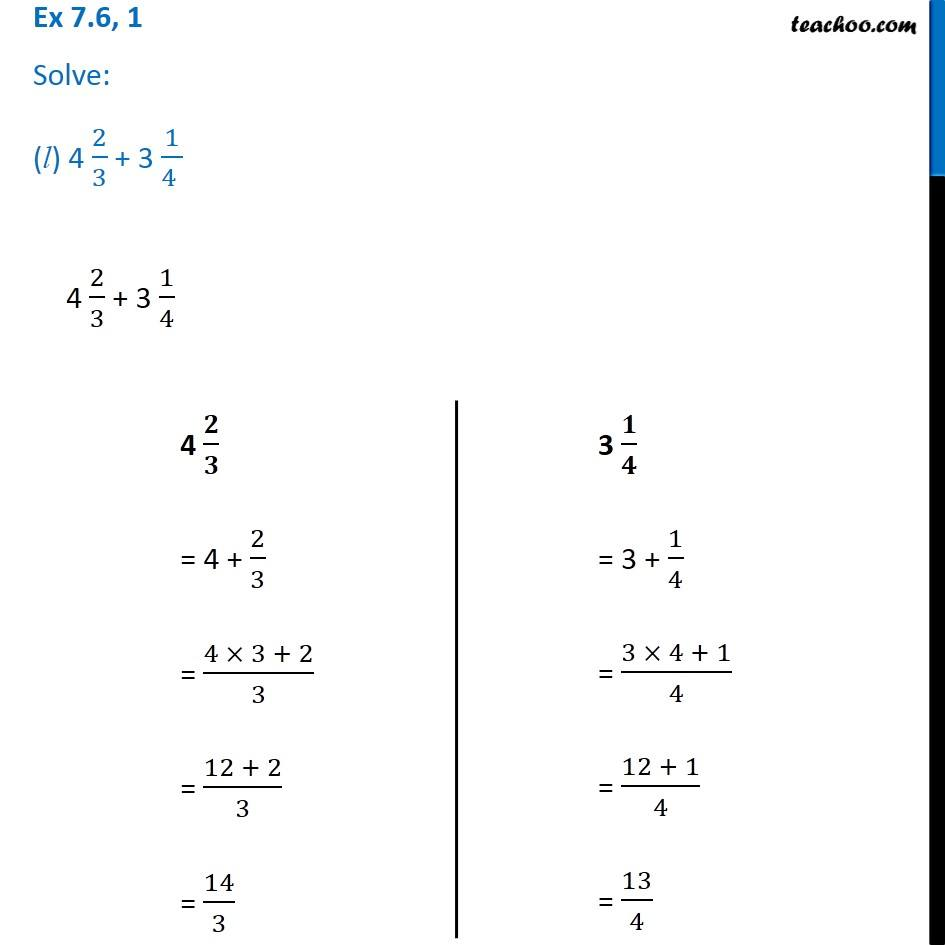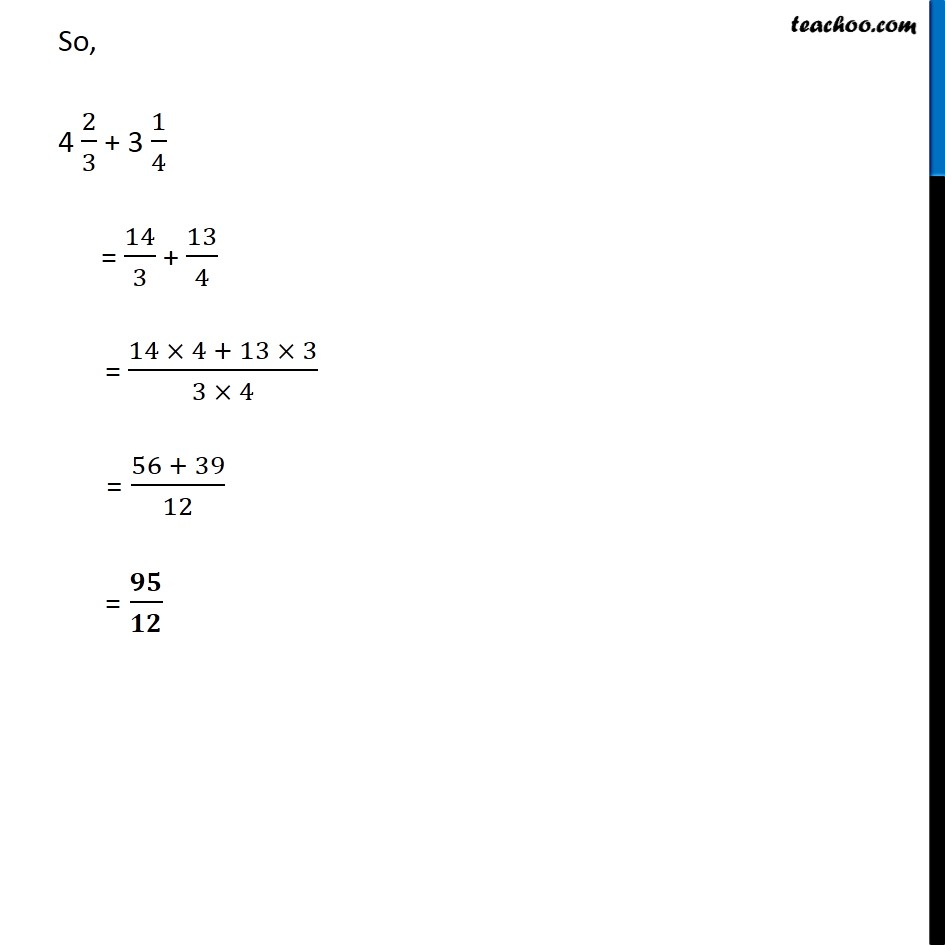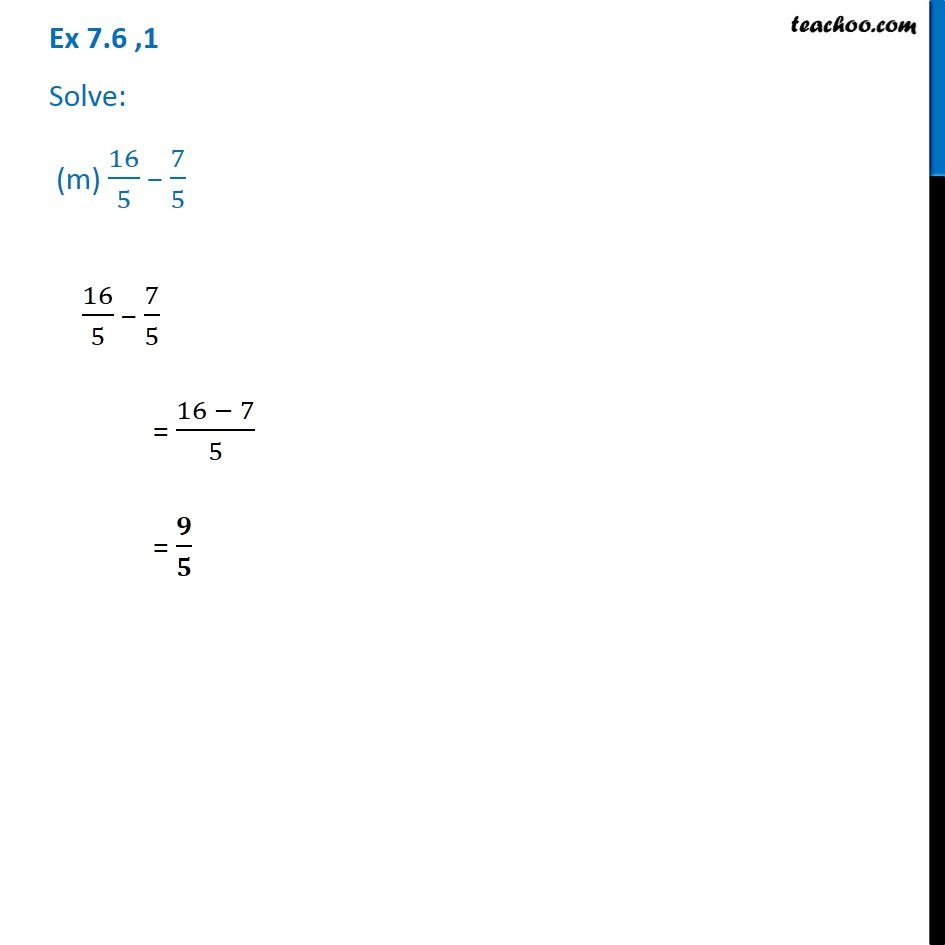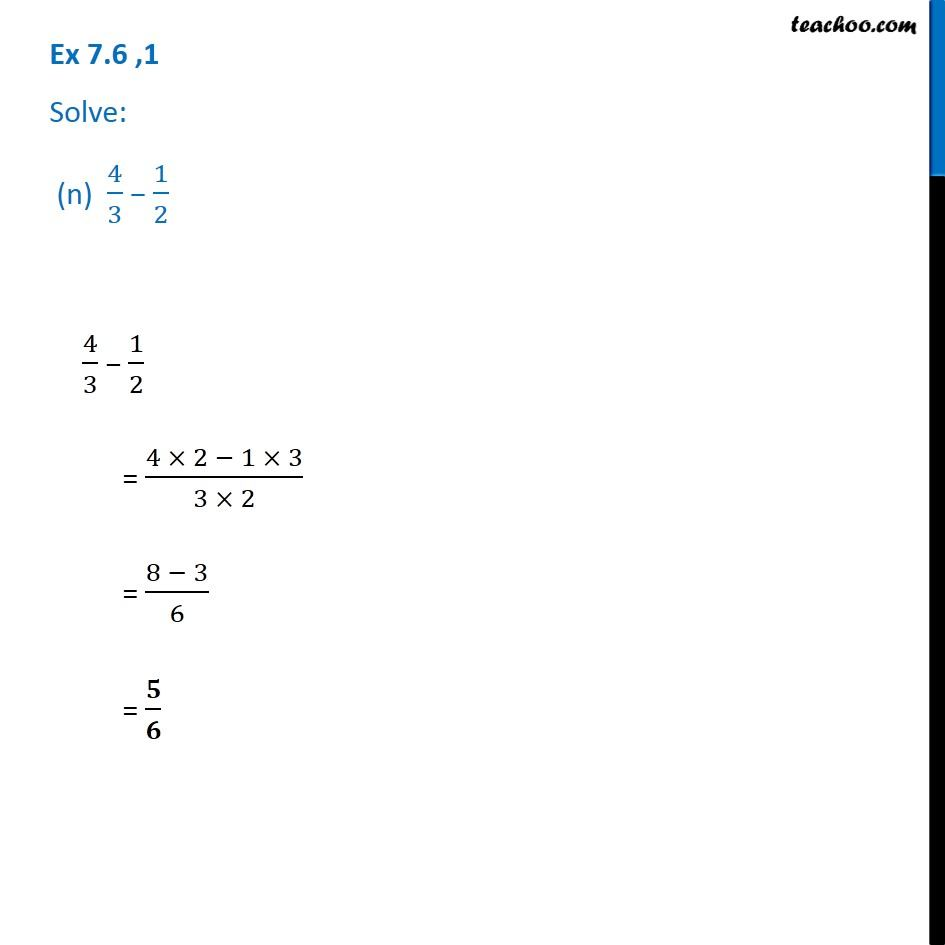1. Chapter 7 Class 6 Fractions
2. Serial order wise
3. Ex 7.6

Transcript

Ex 7.6 ,1 Solve: (a) ( 2)/3 + 1/7 2/5 + 1/7 = (2 7 + 1 3)/(7 3) = (14 + 3)/21 = / Ex 7.6 ,1 Solve: (b) (3 )/10 + 7/15 3/10 + 7/15 = (3 15 + 7 10)/(10 15) = (45 + 70)/150 = 115/150 = / Ex 7.6 ,1 Solve: (c) 4/9 + 2/7 4/9 + 2/7 = (4 7 + 2 9)/(7 9) = (28 + 18)/63 = / Ex 7.6 ,1 Solve: (d) 5/7 + 1/3 5/7 + 1/3 = (5 3 + 1 7)/(7 3) = (15 + 7)/21 = / Ex 7.6 ,1 Solve: (e) 2/5 + 1/6 2/5 + 1/6 = ((2 6) + (1 5))/(6 5) = (12 + 5)/30 = / Ex 7.6 ,1 Solve: (f) 4/2 + 2/3 4/2 + 2/3 = (4 3 + 2 5)/(5 3) = (12 + 10)/15 = / Ex 7.6 ,1 Solve: (g) 3/4 1/3 3/4 1/3 = (3 3 1 4)/(4 3) = (9 4)/12 = / Ex 7.6 ,1 Solve: (h) 5/6 1/3 5/6 1/3 = (5 3 1 6)/(6 3) = (15 6)/18 = 9/18 = 3/6 = / Ex 7.6 ,1 Solve: (i) 2/3 + 3/4 + 1/2 2/3 + 3/4 + 1/2 = 2/3 + (3/4+1/2) = 2/3 + (3 2 + 1 4)/(4 2) = 2/3 + (6 + 4)/8 = 2/3 + 10/8 = 2/3 + 5/4 = (2 4 + 5 3)/(3 4) = (8 + 15)/12 = / Ex 7.6 ,1 Solve: (j) 1/2 + (1 )/(3 ) + 1/6 1/2 + 1/3 + 1/6 = 1/2 + (1/3+1/6) = 1/2 + (1 6 + 1 3)/(6 3) = 1/2 + (6 + 3)/18 = 1/2 + 9/18 = 1/2 + 1/2 = 1/2 + 1/2 = (2 1 + 2 1)/(2 2) = (2 + 2)/4 = 4/4 = 1 Ex 7.6 ,1 Solve: (k) 1 1/3 + 3 2/3 1 1/3 + 3 2/3 1 / = 1 + 1/3 = (1 3 + 1)/3 = (3 + 1)/3 = 4/3 3 / = 3 + 2/3 = (3 3 + 2)/3 = (9 + 2)/3 = 11/3 Now, 1 1/3 + 3 2/3 = 4/3 + 11/3 = 15/3 = 5 Ex 7.6, 1 Solve: (l) 4 2/3 + 3 1/(4 ) Ex 7.6, 1 Solve: (l) 4 2/3 + 3 1/(4 ) Ex 7.6, 1 Solve: (l) 4 2/3 + 3 1/(4 ) 4 2/3 + 3 1/4 4 / = 4 + 2/3 = (4 3 + 2)/3 = (12 + 2)/3 = 14/3 3 / = 3 + 1/4 = (3 4 + 1)/4 = (12 + 1)/4 = 13/4 So, 4 2/3 + 3 1/4 = 14/3 + 13/4 = (14 4 + 13 3)/(3 4) = (56 + 39)/12 = / Ex 7.6 ,1 Solve: (m) 16/5 7/5 16/5 7/5 = (16 7)/5 = / Ex 7.6 ,1 Solve: (n) 4/3 1/2 4/3 1/2 = (4 2 1 3)/(3 2) = (8 3)/6 = /

Ex 7.6

Chapter 7 Class 6 Fractions
Serial order wise